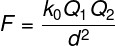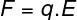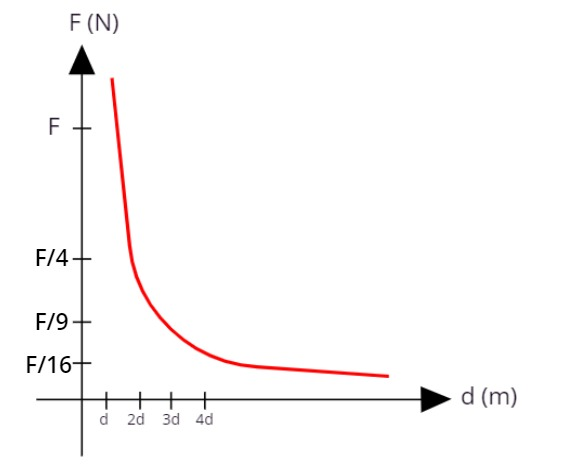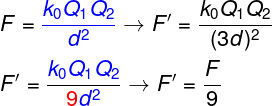Electricity & Megnetism

# Coulomb’s Law

Coulomb ‘s law is the law of physics that allows us to calculate the force of interaction between electric charges. According to this law, the electric force is proportional to the product of the magnitude of two electric charges, as well as inversely proportional to the square of the distance that separates them.

## What does Coulomb’s law say?

According to Coulomb’s law, developed by the French physicist Charles Augustin de Coulomb (1736-1806), it is possible to determine the magnitude of the electric force between two electrically charged particles with charges Q 1 and Q 2 , fixed, located in a vacuum and separated by a distance d, using the following expression:1 and Q 2 – electric charges (C)
0 – electrostatic vacuum constant (k0 = 9,109 N.m²/C²)
d – distance between charges (m)

Coulomb was able to obtain the expression shown above empirically, that is, through experimentation. The experiments that allowed Coulomb to discover the law of attraction and repulsion between electric charges were carried out with a torsion balance, a measuring instrument created by himself that is capable of making precise measurements and which was later used by other researchers, such as Henry Cavendish in the study of universal gravitation .

Also in accordance with Coulomb’s law and also with Newton’s third law , known as the law of action and reaction, charges of like signs tend to repel each other, with forces in the same direction and opposite directions:

Charges of opposite signs, in turn, tend to attract each other with equal forces, and each of these forces also has an opposite direction:

## Coulomb’s Law Formula

As shown earlier, according to Coulomb’s law, the force of interaction between two electrically charged particles, of charges Q 1 and Q 2 , separated in vacuum by a distance d, can be calculated using the following formula:There is also a relationship between the electric force and the electric field . The electric field is the property of electric charges that makes them able to interact with other charges. When an electric charge is placed in a region with an electric field, an electric force acts on it.

The electric force has the same direction as the electric field, unless one of the interacting charges has a negative sign In this case, the direction of the electric force is reversed with respect to the direction of the electric field.

The formula that relates the magnitudes of force and electric field is as follows:E – electric field (N/C)
d – distance to the source of the electric field (m)

## Coulomb’s Law Graph

The graph of the electric force with respect to the distance between two charges is shown in the following figure:Since the electric force is inversely proportional to the square of the distance between the charges , when the distance between the charges is d, the force of interaction between them is F. Doubling the distance between them, the force decreases four times; tripling the distance between the charges, the force between them will be divided by nine, and so on.

## Solved exercises on Coulomb’s law

Question 1 — (EEAR) Two charges are placed in a region where there is electrical interaction between them. When separated by a distance d, the electrical interaction force between them has a magnitude equal to F. By tripling the distance between the charges, the new electrical interaction force in relation to the initial force will be:

a) decreased 3 times.

b) decreased 9 times.

c) increased 3 times.

d) magnified 9 times.

Resolution:

When the charges are at a distance d, the electric force between them is worth F, thus, when we triple the distance between the charges, we will be at a distance 3d. Let’s calculate the value of the new electric force between the charges:According to the calculation, the electrical force between the charges should decrease nine times, so the correct answer is the letter B.

Question 2 — (Udesc) One of the main contributions to the studies on electricity was the precise definition of the nature of the electric force made, mainly, by the works of Charles Augustin de Coulomb (1736-1806). Coulomb performed several experiments to determine the electrical force existing between charged objects, summarizing his conclusions in a relationship we now know as Coulomb’s Law.

Considering Coulomb’s Law, choose the correct alternative.

a) The electric force between two electrically charged bodies is directly proportional to the product of the charges and the square of the distance between these bodies.

b) The electric force between two electrically charged bodies is inversely proportional to the product of the charges and directly proportional to the square of the distance between these bodies.

c) The electric force between two electrically charged bodies is directly proportional to the product of the charges and inversely proportional to the square of the distance between these bodies.

d) The electric force between two electrically charged bodies is directly proportional to the product of the charges and inversely proportional to the distance between these bodies.

e) The electric force between two electrically charged bodies is directly proportional to the distance between these bodies and inversely proportional to the product of the charges.

Resolution:

The correct alternative is the letter C, which is the statement of Coulomb’s law. According to this law, the electric force is proportional to the product of the charges and is inversely proportional to the square of the distance between them.